What is the diameter of a tree around which is 3m 9dm 7cm long rope wrapped? How big is its cross-sectional area?

D =  1.2637 m
S =  1.2542 m2

### Step-by-step explanation: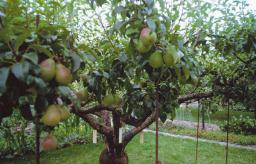Did you find an error or inaccuracy? Feel free to write us. Thank you!Tips to related online calculators
Do you want to convert length units?

#### You need to know the following knowledge to solve this word math problem:

We encourage you to watch this tutorial video on this math problem:

## Related math problems and questions:Miro stands under a tree and watching its shadow and shadow of the tree. Miro is 180 cm tall and its shade is 1.5 m long. The shadow of the tree is three times as long as Miro's shadow. How tall is the tree in meters?
• Chimney and treeCalculate the height of the factory chimney, which casts a shadow 6.5 m long in the afternoon. At the same time, a 6 m high tree standing near it casts a shadow 25 dm long.
• Winch drum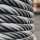Originally an empty winch drum with a diameter of 20 cm and a width of 30 cm on the rescue car, he started winding a rope with a thickness of 1 cm beautifully from edge to edge. The winch stopped after 80 turns. It remains to spin 3.54m of rope (without hTree perpendicular to the horizontal surface has a shadow 8.32 meters long. At the same time meter rod perpendicular to the horizontal surface has shadow 64 cm long. How tall is tree?A 2-meter rod casts a shadow 3.2 m long. How high is a tree with a shadow of 14.4 m?
• BaseBase of building is circle with diameter 25 m. Calculate the circumference of a circular trench witch diameter is 41 cm wider than the diameter of the base.
• Diameter of a cylinderThe diameter of the cylinder is 42 cm. How many times does the cylinder turn on a 66m long track?
• Round table tablecloth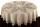The round table has an area of 50.24 dm2. Calculate the circular tablecloth diameter, if it extends beyond the edge of the table by 30 cm.
• Pulley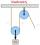On wheels with a diameter of 40 cm is fixed rope with the load. Calculate how far is load lifted when the wheel turns 7 times?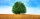The shadow of a 1 m high pole thrown on a horizontal plane is 0.8 m long. At the same time, the shadow of a tree thrown on a horizontal plane is 6.4 m. Determine the height of the tree.
• A rope 2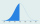A rope that is 6 meters long will be cut into 24 pieces that are all of the same length. What will be the length of each piece in centimeters? (100 cm = 1 m)
• Pine woodFrom a trunk of pine 6m long and 35 cm in diameter with a carved beam with a cross-section in the shape of a square so that the square had the greatest content area. Calculate the length of the sides of a square. Calculate the volume in cubic meters of lu
• Rope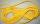Peter has a long rope 2 4/5 meter. Martin has a rope 20 cm longer. What distance ropes achieved if the boys link ropes?
• Diameter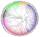The diameter of a circular plot is 14 dm. Find the circumference and area.
• Bicycle wheelA bicycle wheel has a diameter of 60 cm. Approximately how many times does the wheel rotate at 2.5 km long trip?
• The chimney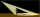The chimney casts a shadow 45 meters long. The one-meter-long rod standing perpendicular to the ground has a shadow 90 cm long. Calculate the height of the chimney.
• Vertical rodThe vertical one-meter-long rod casts a shadow 150 cm long. Calculate the height of a column whose shadow is 36 m long at the same time.